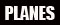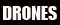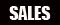This thread is privately moderated by Miami Mike, who may elect to delete unwanted replies.
Jan 02, 2017, 11:33 AM
Healthy suntan is an oxymoron.
Discussion

# Taranis: How the Data Flows

Here are some examples of experiments you can do to determine the order in which data is processed by the OpenTX Inputs and Mixes pages:

Experimental Testing of OpenTX Data Flow
• Set up a new model memory for testing.
1. Create a single input on the Inputs page.
Code:
`[I1] Ail Weight(+100%)`
2. Create a single mix on the Mixes page.
Code:
`[I1]Ail Weight (+100%)`
3. On the Outputs page, ensure that all channels are set to the defaults of
• Subtrim = 0.0%
• Min = -100%
• Max = +100%
• Direction = ---
• Curve = ---
4. Test the basic setup.
• Start Companion simulator and move the aileron stick left and right. Observe that the CH01 indicator moves left and right from -100% to +100%.
• Exit the simulator.
5. Create a special test curve.
• On the Curves page, create a 7-level, 12-point, stair-step curve.
• Select Curve 1.
• Select 12 points.
• Select Custom X.
• Enter these values:• You are now set up to perform experiments to determine the order in which data are processed in the Inputs and Mixes pages by OpenTX.
• Testing the Data Flow in Mixes
1. Experiment #1: In Mixes, which is processed first, Weight or Curve?
• On the Mixes page, copy the mix from CH01 to CH02.
• Add Curve 1 to the CH02 mix:
Code:
`CH02 [I1]Ail Weight(+100%) Curve(1)`
• Start Companion simulator and move the aileron stick left and right. Observe how the CH02 indicator steps through a range of 7 discrete levels as it moves left and right from -100% to +100%.
• Exit the simulator.
• Reduce the weight in the CH02 mix:
Code:
`CH02 [I1]Ail Weight(+50%) Curve(1)`
• Start Companion simulator and move the aileron stick left and right.
• Observe that the CH02 indicator travel is now restricted to the range of -50% to +50%, as expected.
• Count how many discrete levels the CH02 indicator assumes as it steps through its range of -50% to +50%.
• If Curve is processed before Weight then we should still see CH02 transitioning between 7 discrete levels, although the range will be restricted to -50% to +50%.
• But if Weight is processed before Curve, we should see that portions of the beginning and end of the curve are being trimmed away, resulting in CH02 transitioning between fewer than 7 discrete levels.
• Experiment #2: Reproduce the result we would expect to see if Weight is processed before Curve.
• Copy the mix from CH01 to CH10.
Code:
`CH10 [I1]Ail Weight(+100%)`
• Change the Source of the CH02 mix from [I1]Ail to CH10:
Code:
`CH02 CH10 Weight (+100%) Curve(1)`
• Start Companion simulator and move the aileron stick left and right. Observe how the CH02 indicator steps through a range of 7 discrete levels as it moves left and right from -100% to +100%, just as it did before.
• Exit the simulator.
• Now reduce Weight in the CH10 mix to 50%:
Code:
`CH10 [I1]Ail Weight(+50%)`
Now we've created a setup where we can be confident that Weight is being processed before Curve, and with reduced weight we can expect to see a reduced number of curve steps.
• Start Companion simulator and move the aileron stick left and right.
• Count how many discrete levels the CH02 indicator assumes as it steps through its range of -50% to +50%.
• Exit the simulator.
• Experiment #3: Reproduce the result we would expect to see if Curve is processed before Weight.
• Since this is a continuation of Experiment #2, we'll make some alterations to the mixes already set up for the previous experiment.
• Remove the curve from CH02 and reduce Weight to 50%, i.e change CH02 from this:
Code:
`CH02  CH10 Weight(+100%) Curve(1)`
to this:
Code:
`CH02  CH10 Weight(+50%)`
• Add the curve to CH10 and increase Weight to 100%, i.e. change CH10 from this:
Code:
`CH10  [I1]Ail Weight(+50%)`
to this:
Code:
`CH10  [I1]Ail Weight(+100%) Curve(1)`
Now we've created a setup where we can be confident that Curve is being processed before Weight, and with reduced weight we can expect to see all 7 curve steps across a reduced range.
• Start Companion simulator and move the aileron stick left and right.
• Count how many discrete levels the CH02 indicator assumes as it steps through its range of -50% to +50%.
• Exit the simulator.
• Experiment #4: In mixes, when is the "slow" function processed?
• Set up the following mixes:
Code:
`CH01  [I1]Ail Weight(+100%) Curve(1) Slow (u5:d5)`
Code:
`CH02  CH10 Weight (+100%) Slow(u5:d5)`
Code:
`CH10  [I1]Ail Weight(+100) Curve(1)`
• If "slow" is processed at the end of the mix, after Curve and Weight, then we would expect CH01 to move slowly from one position to the next and CH02 to jump quickly between levels.
• But if "slow" is processed at the beginning of the mix, before Curve and Weight (or at least before Curve), then we would expect CH01 to jump quickly between levels and CH02 to move slowly from one position to the next.

Now let's do one more, this time with Inputs:

2. Testing the Data Flow in Inputs
• Experiment #5: In Inputs, which is processed first, Weight or Curve?
• Restore the CH01 mix to what we started with:
Code:
`CH01  [I1] Ail Weight(+100%)`
• Delete the other mixes.
• Switch to the Inputs page and add the stair step curve to the [i1]Ail input:
Code:
`[I1]Ail  Ail Weight(+100%) Curve(1)`
• Test with Companion simulator.
• Exit the simulator.
• Now reduce the Weight to 50%:
Code:
`[I1]Ail  Ail Weight(+50%) Curve(1)`
• Test again with Companion simulator
• Just as in the mix test,
• If Curve is processed before Weight then we should still see CH01 assume 7 discrete levels, although the range will be restricted to -50% to +50%.
• But if Weight is processed before Curve, we should see that portions of the beginning and end of the curve are being trimmed away, resulting in CH01 assuming fewer than 7 discrete levels.

I could just list my results, but I wouldn't want to spoil anybody's fun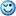, and I wouldn't expect anyone to take my word for it. For now, I suggest you try these experiments yourself. They may help you gain a better understanding of how OpenTX works.
Last edited by Miami Mike; Jan 03, 2017 at 03:30 PM.
 Sign up now to remove ads between postsJan 03, 2017, 09:04 AM Froggy RC Pilot Hi Mike, Just one comment, in the data flow shown in my diagrams (FrSky Taranis - OpenTX data flow diagrams), the weight and curve are going through a multiplication. One of the main properties of multiplication is the commutative property, so Weight x Curve is the same than Curve x Weight.
 Jan 03, 2017, 09:32 AM Healthy suntan is an oxymoron. No it's not. "Curve" is a function and can't be mathematically treated as a factor. My experiments #2 and #3 prove that. Latest blog entry: OpenTX: Circling the Square
 Jan 03, 2017, 09:59 AM Froggy RC Pilot Here is my explanation: experiment #1 → Output = Input x ( 0.5 x Curve 1). Which is the same thing than Output = Input x ( Curve 1 x 0.5) experiment #2 → Output 1 = Input x 0.5, then Output 2 = Output 1 x Curve 1. experiment #3 → Output 1 = Input x Curve, then Output 2 = Output 1 x Weight. In you experiment #1, you do Input x (Weight x Curve) or Input x (Curve x Weight). In you experiment #2, you do (Input x Weight) x Curve. In you experiment #3, you do (Input x Curve) x Weight. So you don't get the same result because you don't apply the same calculation. Don't forget than your Curve is a non-linear and discontinuous function. So the brackets are very important.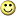Last point, this kind of Curve is definitely not a factor.
Jan 03, 2017, 10:27 AM
Healthy suntan is an oxymoron.
Quote:
 Originally Posted by LapinFou Last point, this kind of Curve is definitely not a factor.
Yes, that's what I'm trying to tell you. I think it might help if you'd stop writing your expressions as if they were.
Latest blog entry: OpenTX: Circling the Square
 Jan 03, 2017, 10:34 AM Froggy RC Pilot OK. I misread your previous comment. I read "[...] and can be mathematically treated as a factor [...]" instead "[...] and can't be mathematically treated as a factor [...]". My mistake. After some thoughts about your experiments, you're right writing Input x (Curve x Weight) is closer to the reality. I will update my diagrams.
 Jan 03, 2017, 11:15 AM Froggy RC Pilot My diagrams have been updated. Thanks to you for your very instructive experiments.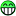Jan 03, 2017, 11:51 AM Healthy suntan is an oxymoron. You're welcome! Please keep in mind that those five experiments were only examples illustrating one of what may be several possible methods of testing data flow. It wasn't by any means a complete analysis. I still don't know where Offset, Switch, Flight Modes, or Delay belong in the Mixes diagram, or where Stick Side, Offset, Switch, or Flight Modes belong in the Inputs diagram. And possibly most important of all, I haven't yet investigated the Output/Servos data flow at all. You might want to consider experimenting with those other aspects of your diagrams. Latest blog entry: OpenTX: Circling the Square
 Jan 03, 2017, 01:05 PM Froggy RC Pilot Yes, I understand. When I created my diagrams, I've done some extensive experiments and many corrections. So, about the offset, switch, FM, etc... I'm pretty sure that is correct. Moreover I exchanged a lot with Kilrah (OTX member) about those diagrams.I misses the Weight/Curve thing because I assumed than the curve was a linear function. Obviously, this was a bad guess!Jan 03, 2017, 04:46 PM Healthy suntan is an oxymoron. And the Slow option? Try this square curve as Curve(2) :and set up these mixes: Code: `CH01 [I1]Ail Weight (+100%) Slow(u5:d5)` Code: `CH02 [I1]Ail Weight (+100%) Curve(2) Slow(u5:d5)` If Slow happens at the end of the mix then there shouldn't be any way to make the output jump instantaneously from one extreme to the other, yet that's what happens with CH02. It's clear here that the Slow option is slowing down changes in the input before the curve, not the output after the curve. Edit: I had a hastily cooked up test for Delay here but I changed my mind and decided to rethink it. I've asked Kilrah to join our discussion here. Last edited by Miami Mike; Jan 04, 2017 at 06:00 PM.
 Jan 03, 2017, 05:12 PM Froggy RC Pilot I will have a look tomorrow (I'm currently in bed with my tablet), however there is a known issue. We had a long discussion about the output glitching when a curve, slow parameter and switch are used at the same time. More info here (sorry it is in French): http://frskytaranis.forumactif.org/t...ralenti-courbe
 Jan 03, 2017, 05:15 PM Happy FPV flyer Maybe at some point the easiest is to read the code? https://github.com/opentx/opentx/blo.../src/mixer.cpp
Jun 11, 2017, 04:55 PM
Registered User
Quote:
 Originally Posted by Kilrah Maybe at some point the easiest is to read the code? https://github.com/opentx/opentx/blo.../src/mixer.cpp
From the looks of things, the master branch is no longer.

For 2.2 the file is here: https://github.com/opentx/opentx/blo.../src/mixer.cpp

Miami Mike,

Thanks for putting this together.

My assumption is that this is for 2.2 since Kilrah was referring to master.

 Thread Tools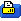Show Printable Version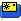Email this Page